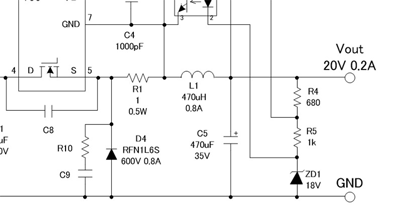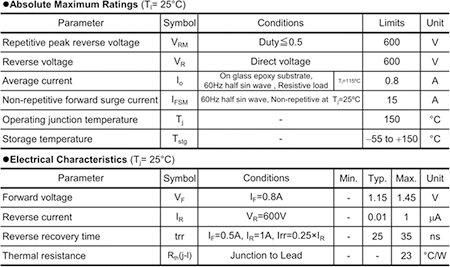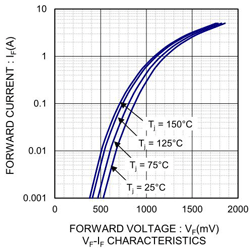Technical Information Site of Power Supply Design

• Basic Knowledge
• AC/DC
• Design Examples of Non-isolated Buck Converters
• Selecting Critical Components: Output Rectifying Diode D4

2018.02.08 AC/DC

# Selecting Critical Components: Output Rectifying Diode D4

Design Examples of Non-isolated Buck Converters

This time, we will explain selection of the output rectifying diode.

Output Rectifying Diode D4

The output rectifying diode is also called a catch diode or a freewheel diode. In a synchronous rectifying design, this diode is replaced with a transistor, which is called a low-side transistor or the like.

As indicated in the circuit diagram on the right, the output diode D4 is connected from the output to GND. When the high-side MOSFET turns off, energy stored in the inductor is output through D4.Calculation of Output Diode Component Values

The output rectifying diode turns on and off at the switching frequency, and so a fast recovery diode capable of high-speed switching is used. The rated voltage and losses need to be considered.

The reverse voltage applied to the output diode should be as follows, including a margin.

Vdr = VIN (max)÷0.7 = 372V÷0.7 = 531V →　600V adopted

As a rough estimate, diode losses are as follows.

Pd = VF×Iout = 1V×0.2A = 0.2W

As the diode, a RFN1L6S is used, as specified in the circuit diagram.

The following are a table of specifications for the fast recovery diode RFN1L6S and the VF-IF characteristic. This time, there is a circuit example, and so there is no need to actually search for a diode; but let us use the opportunity to study this RFN1L6S, on the assumption that we have come across it as a diode with a 600 V rated voltage that can pass 0.2 A or more current.As the rated voltage, 600 V is the maximum rating for both VR and VRM, agreeing with the calculated value. IO is 0.8 A, and so in terms of performance there is a considerable margin relative to an Iout of 0.2 A, but this much of a margin may be desirable considering the allowable power. Moreover, there may not be component varieties that are an exact match to the design specs. Rather, exact matches are actually rare. In any case, it is necessary to make some compromises, with approximations made in favor of a larger margin.

Calculations were performed with 1 V assumed for VF, but the specs indicate that the maximum values is 1.45 V and the typical value is stipulated to be 1.15 V; the VFｰIF characteristic is shown in order to reassure those persons who are wondering whether calculations assuming 1 V for VF are accurate. As a condition for VF, IF is taken to be 0.8 A. In actual use, IF is 0.2 A, and upon determining VF from the graph for an IF of 0.2 A, we see that even under the worst temperature conditions, VF is below 1 V. In other words, these calculations were performed using numerical values close to actual conditions.

How large of a margin to secure will depend on empirical rules. If the margin is too large, the design becomes excessive, so that costs and size may be bloated. Decisions here are not easy, and as in many other areas, will depend on accumulated experience.

We have spoken of empirical rules, but it is essential that losses and junction temperatures be checked under conditions of actual use.

#### Key Points:

・Normally, a device capable of fast switching is used as the output rectifying diode. Here, a fast recovery diode is used.

・An output rectifying diode is in essence selected based on consideration of the rated voltage and losses.